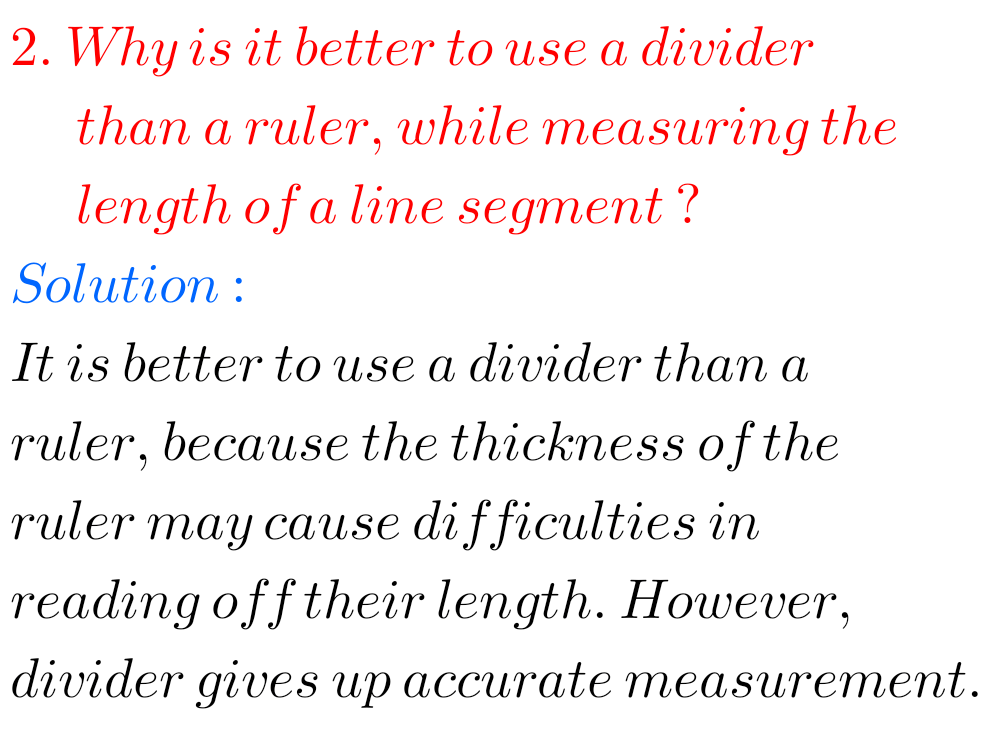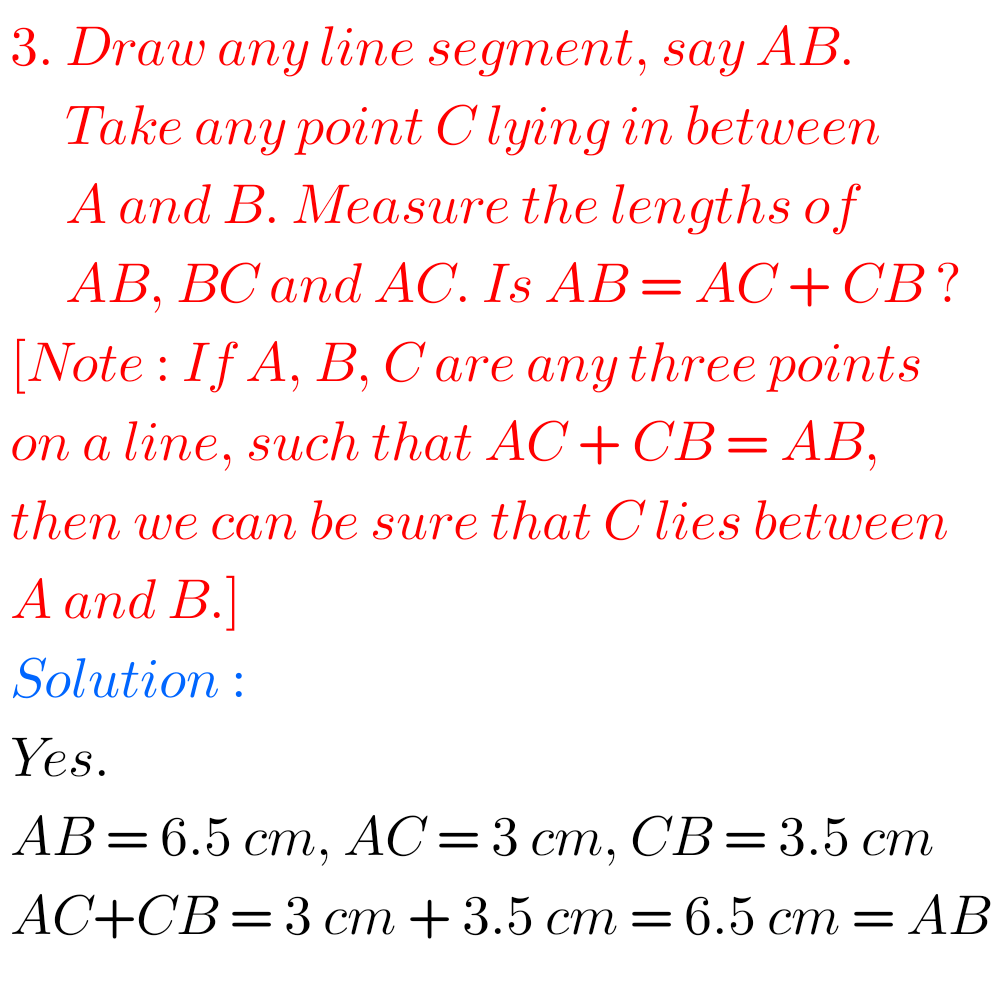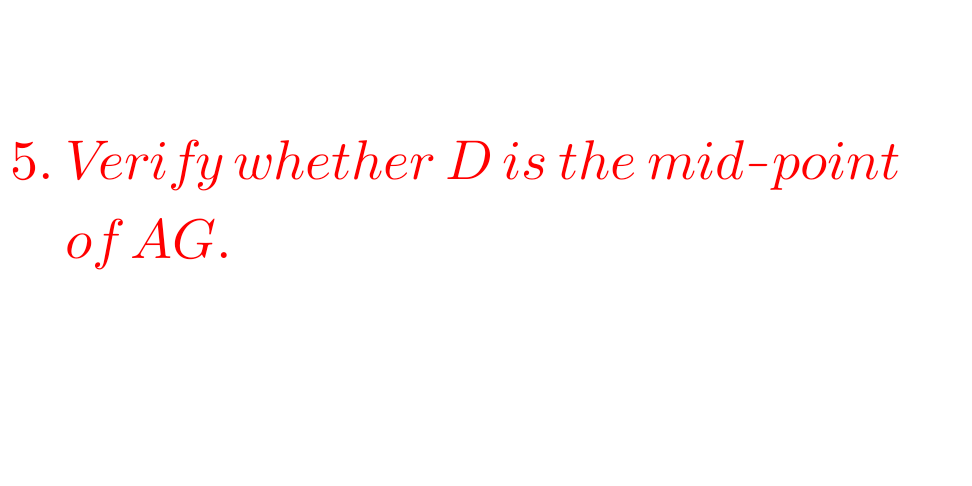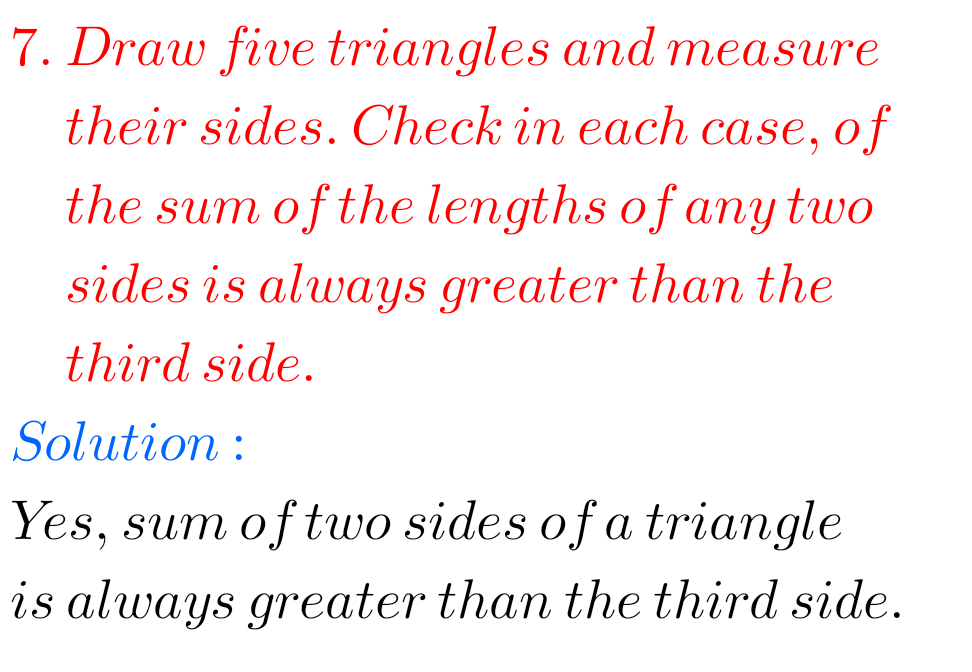# 6th class maths solutions for chapter 5 Understanding Elementary Shapes exercise 5.1

Ncert Maths Class 6 chapter 5 Understanding Elementary Shapes Exercise 5.1 solutions are given.

Study the textbook lesson Understanding Elementary Shapes very well.

Observe the example problems and solutions given in the textbook.

Observe the solutons and try them in your own method.

You can see

Ncert maths solutions for class 6
Understanding elementary shapes

Exercise 5.1

Exercise 5.2

Exercise 5.3

Exercise 5.4

Exercise 5.5

Exercise 5.6

Exercise 5.7

Exercise 5.8

Exercise 5.9

M

# Exercise 5.1 chapter 5 class 6 Ncert Maths

Chapter – 5

Understanding Elementary Shapes

Exercise 5.1F

## Maths solutions Ncert Class 6 Exercise 5.1 Understanding Elementary ShapesFF

## Chapter 5 Understanding  Elementary Shapes Exercise 5.1 solutions Ncert class 6 MathsF

M

Note : Observe the solutions and try them in your own method.